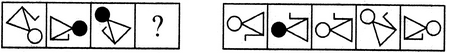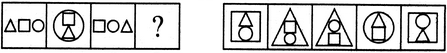# Non Verbal Reasoning - Analogy

Exercise : Analogy - Section 1
Directions to Solve

Each of the following questions consists of two sets of figures. Figures A, B, C and D constitute the Problem Set while figures 1, 2, 3, 4 and 5 constitute the Answer Set. There is a definite relationship between figures A and B. Establish a similar relationship between figures C and D by selecting a suitable figure from the Answer Set that would replace the question mark (?) in fig. (D).

11.

Select a suitable figure from the Answer Figures that would replace the question mark (?).(A)     (B)      (C)     (D)                  (1)      (2)      (3)      (4)      (5)
1
2
3
4
5
Explanation:
The trapezium gets vertically inverted and move to the middle right position; the pin rotates 90oCW and moves to the lower-right position; the third element rotates 135oACW.

12.

Select a suitable figure from the Answer Figures that would replace the question mark (?).(A)     (B)      (C)     (D)                  (1)      (2)      (3)      (4)      (5)
1
2
3
4
5
Explanation:
The figure rotates 45oACW; the circle changes colour (turns black if initially white and vice-versa). The 'L'-shaped element shifts to the other side of the main figure.

13.

Select a suitable figure from the Answer Figures that would replace the question mark (?).(A)     (B)      (C)     (D)                  (1)      (2)      (3)      (4)      (5)
1
2
3
4
5
Explanation:
The rightmost element enlarges to become the outer element; the leftmost element becomes the inner-lower element and the middle element becomes the inner-upper element.

14.

Select a suitable figure from the Answer Figures that would replace the question mark (?).(A)     (B)      (C)     (D)                  (1)      (2)      (3)      (4)      (5)
1
2
3
4
5
Explanation:
The figure gets laterally inverted.

15.

Select a suitable figure from the Answer Figures that would replace the question mark (?).